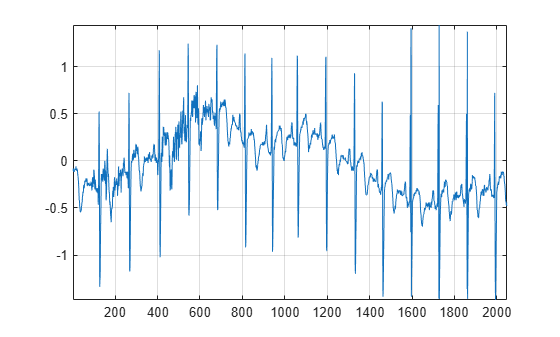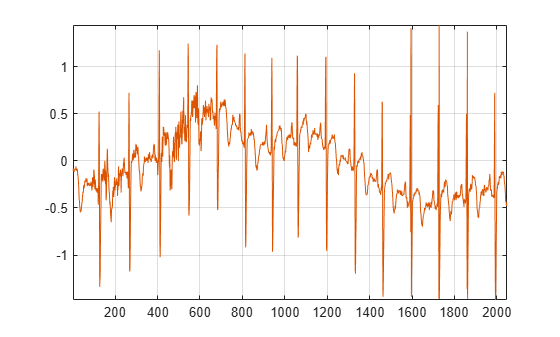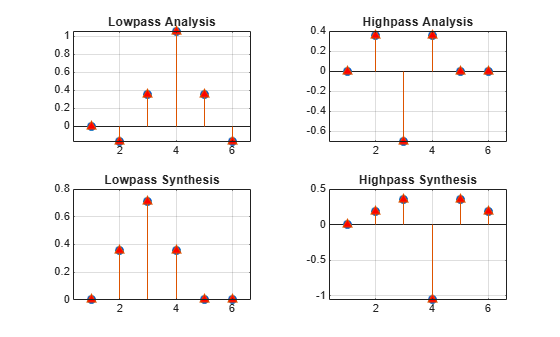Main Content

# idwpt

Multisignal 1-D inverse wavelet packet transform

## Syntax

``xrec = idwpt(wpt,l)``
``xrec = idwpt(wpt,l,wname)``
``xrec = idwpt(wpt,l,LoR,HiR)``
``xrec = idwpt(___, 'Boundary',ExtensionMode)``

## Description

````xrec = idwpt(wpt,l)` inverts the discrete wavelet packet transform (DWPT) of the terminal node wavelet packet tree `wpt` using the bookkeeping vector `l`. The `idwpt` function assumes that you obtained `wpt` and `l` using `dwpt` with the `fk18` wavelet and default settings.If the input to `dwpt` was one signal, `xrec` is a column vector. If the input was a multichannel signal, `xrec` is a matrix, where each matrix column corresponds to a channel.```
````xrec = idwpt(wpt,l,wname)` uses the wavelet specified by `wname` to invert the DWPT. `wname` must be recognized by `wavemngr`. The specified wavelet must be the same wavelet used to obtain the DWPT.```

example

````xrec = idwpt(wpt,l,LoR,HiR)` uses the scaling (lowpass) filter, `LoR`, and wavelet (highpass) filter, `HiR`. The synthesis filter pair `LoR` and `HiR` must be associated with the same wavelet used in the DWPT.```

example

````xrec = idwpt(___, 'Boundary',ExtensionMode)` specifies the mode to extend the signal. `ExtensionMode` can be either `'reflection'` (default) and `'periodic'`. By setting `ExtensionMode` to `'periodic'` or `'reflection'`, the wavelet packet coefficients at each level are extended based on the modes `'per'` or `'sym'` in `dwtmode`, respectively. `ExtensionMode` must be the same mode used in the DWPT.```

## Examples

collapse all

This example shows how to perform the inverse wavelet packet transform using synthesis filters.

Obtain the DWPT of an ECG signal using `dwpt` with default settings.

```load wecg [wpt,l] = dwpt(wecg); ```

By default, `dwpt` uses the `fk18` wavelet. Obtain the synthesis (reconstruction) filters associated with the wavelet.

```[~,~,lor,hir] = wfilters('fk18'); ```

Invert the DWPT using the synthesis filters and demonstrate perfect reconstruction.

```xrec = idwpt(wpt,l,lor,hir); norm(wecg-xrec,'inf') ```
```ans = 4.9236e-11 ```

Obtain the DWPT of an ECG signal using `dwpt` and periodic extension.

```load wecg [wpt,l] = dwpt(wecg,'Boundary','periodic');```

By default, `idwpt` uses symmetric extension. Invert the DWPT using periodic and symmetric extension modes.

```xrecA = idwpt(wpt,l,'Boundary','periodic'); xrecB = idwpt(wpt,l);```

Demonstrate perfect reconstruction only when the extension modes of the forward and inverse DWPT agree.

`fprintf('Periodic/Periodic : %f\n',norm(wecg-xrecA,'inf'))`
```Periodic/Periodic : 0.000000 ```
`fprintf('Periodic/Symmetric: %f\n',norm(wecg-xrecB,'inf'))`
```Periodic/Symmetric: 1.477907 ```

This example shows how to take an expression of a biorthogonal filter pair and construct lowpass and highpass filters to produce a perfect reconstruction (PR) pair in Wavelet Toolbox™.

The LeGall 5/3 filter is the wavelet used in JPEG2000 for lossless image compression. The lowpass (scaling) filters for the LeGall 5/3 wavelet have five and three nonzero coefficients respectively. The expressions for these two filters are:

`${H}_{0}\left(z\right)=1/8\left(-{z}^{2}+2z+6+2{z}^{-1}-{z}^{-2}\right)$`

`${H}_{1}\left(z\right)=1/2\left(z+2+{z}^{-1}\right)$`

Create these filters.

```H0 = 1/8*[-1 2 6 2 -1]; H1 = 1/2*[1 2 1];```

Many of the discrete wavelet and wavelet packet transforms in Wavelet Toolbox rely on the filters being both even-length and equal in length in order to produce the perfect reconstruction filter bank associated with these transforms. These transforms also require a specific normalization of the coefficients in the filters for the algorithms to produce a PR filter bank. Use the `biorfilt` function on the lowpass prototype functions to produce the PR wavelet filter bank.

`[LoD,HiD,LoR,HiR] = biorfilt(H0,H1);`

The sum of the lowpass analysis and synthesis filters is now equal to $\sqrt{2}$.

`sum(LoD)`
```ans = 1.4142 ```
`sum(LoR)`
```ans = 1.4142 ```

The wavelet filters sum, as required, to zero. The L2-norms of the lowpass analysis and highpass synthesis filters are equal. The same holds for the lowpass synthesis and highpass analysis filters.

Now you can use these filters in discrete wavelet and wavelet packet transforms and achieve a PR wavelet packet filter bank. To demonstrate this, load and plot an ECG signal.

```load wecg plot(wecg) axis tight grid on```Obtain the discrete wavelet packet transform of the ECG signal using the LeGall 5/3 filter set.

`[wpt,L] = dwpt(wecg,LoD,HiD);`

Now use the reconstruction (synthesis) filters to reconstruct the signal and demonstrate perfect reconstruction.

```xrec = idwpt(wpt,L,LoR,HiR); plot([wecg xrec]) axis tight, grid on;````norm(wecg-xrec,'Inf')`
```ans = 3.3307e-15 ```

You can also use this filter bank in the 1-D and 2-D discrete wavelet transforms. Read and plot an image.

```im = imread('woodsculp256.jpg'); image(im); axis off;```Obtain the 2-D wavelet transform using the LeGall 5/3 analysis filters.

`[C,S] = wavedec2(im,3,LoD,HiD);`

Reconstruct the image using the synthesis filters.

```imrec = waverec2(C,S,LoR,HiR); image(uint8(imrec)); axis off;```The LeGall 5/3 filter is equivalent to the built-in `'bior2.2'` wavelet in Wavelet Toolbox. Use the `'bior2.2'` filters and compare with the LeGall 5/3 filters.

```[LD,HD,LR,HR] = wfilters('bior2.2'); subplot(2,2,1) hl = stem([LD' LoD']); hl(1).MarkerFaceColor = [0 0 1]; hl(1).Marker = 'o'; hl(2).MarkerFaceColor = [1 0 0]; hl(2).Marker = '^'; grid on title('Lowpass Analysis') subplot(2,2,2) hl = stem([HD' HiD']); hl(1).MarkerFaceColor = [0 0 1]; hl(1).Marker = 'o'; hl(2).MarkerFaceColor = [1 0 0]; hl(2).Marker = '^'; grid on title('Highpass Analysis') subplot(2,2,3) hl = stem([LR' LoR']); hl(1).MarkerFaceColor = [0 0 1]; hl(1).Marker = 'o'; hl(2).MarkerFaceColor = [1 0 0]; hl(2).Marker = '^'; grid on title('Lowpass Synthesis') subplot(2,2,4) hl = stem([HR' HiR']); hl(1).MarkerFaceColor = [0 0 1]; hl(1).Marker = 'o'; hl(2).MarkerFaceColor = [1 0 0]; hl(2).Marker = '^'; grid on title('Highpass Synthesis')```## Input Arguments

collapse all

Terminal node wavelet packet tree, specified as a cell array. `wpt` is the output of `dwpt` with the `'FullTree'` value set to `false`.

Example: `[wpt,l] = dwpt(X,'Level',3,'FullTree',false)` returns the terminal node wavelet packet tree of the three-level wavelet packet decomposition of `X`.

Data Types: `single` | `double`

Bookkeeping vector, specified as a vector of positive integers. The vector `l` is the output of `dwpt`. The bookkeeping vector contains the length of the input signal and the number of coefficients by level, and is required for perfect reconstruction.

Data Types: `single` | `double`

Wavelet to use in the inverse DWPT, specified as a character vector or string scalar. `wname` must be recognized by `wavemngr`. The specified wavelet must be the same wavelet used to obtain the DWPT.

You cannot specify both `wname` and a filter pair, `LoD` and `HiD`.

Example: `xrec = idwpt(wpt,l,"sym4")` specifies the `sym4` wavelet.

Wavelet synthesis (reconstruction) filters to use in the inverse DWPT, specified as a pair of real-valued vectors. `LoR` is the scaling (lowpass) synthesis filter, and `HiR` is the wavelet (highpass) synthesis filter. The synthesis filter pair must be associated with the same wavelet as used in the DWPT. You cannot specify both `wname` and a filter pair, `LoR` and `HiR`. See `wfilters` for additional information.

Note

`idwpt` does not check that `LoR` and `HiR` satisfy the requirements for a perfect reconstruction wavelet packet filter bank. See PR Biorthogonal Filters for an example of how to take a published biorthogonal filter and ensure that the analysis and synthesis filters produce a perfect reconstruction wavelet packet filter bank using `idwpt`.

Wavelet packet transform boundary handling, specified as `'reflection'` or `'periodic'`. When set to `'reflection'` or `'periodic'`, the wavelet packet coefficients are extended at each level based on the `'sym'` or `'per'` mode in `dwtmode`, respectively. `ExtensionMode` must be the same mode used in the DWPT. If unspecified, `ExtensionMode` defaults to `'reflection'`.

 Wickerhauser, Mladen Victor. Adapted Wavelet Analysis from Theory to Software. Wellesley, MA: A.K. Peters, 1994.

 Percival, D. B., and A. T. Walden. Wavelet Methods for Time Series Analysis. Cambridge, UK: Cambridge University Press, 2000.

 Mesa, Hector. “Adapted Wavelets for Pattern Detection.” In Progress in Pattern Recognition, Image Analysis and Applications, edited by Alberto Sanfeliu and Manuel Lazo Cortés, 3773:933–44. Berlin, Heidelberg: Springer Berlin Heidelberg, 2005. https://doi.org/10.1007/11578079_96.

## SupportGet trial now